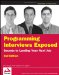# Chapter 9: Object-Oriented Programming

These days, most programming is done using an object-oriented (OO) language such as Java, C#, or C++. Even JavaScript, though not an OO language, supports some features of OO programming through prototype objects and the clever use of function definitions. As such, it’s important to have a good grasp of fundamental OO principles, so this entire chapter is devoted to the topic.

## Fundamentals

Although not widely used until the turn of the century, object-oriented programming’s roots date back several decades to languages such as Simula and Smalltalk. OO programming has been the subject of much academic research and debate, especially since the widespread adoption of OO programming languages by practicing developers.

### Classes and Objects

There are many different ways and no clear consensus on how to describe and define object orientation as a programming technique, but all of them revolve around the notions of classes and objects. A class is an abstract definition of something that has attributes (sometimes called properties or states) and actions (capabilities or methods). An object is a specific instance of a class that has its own state separate from any other object instance. Here’s a class definition for Point, which is a pair of integers that represents the x and y values of a point in a Cartesian coordinate system:

` public class Point {     private int x;     private int y;     public Point( int x, int y ){         this.x = x;         this.y = y;     }     public Point( Point other ){         x = other.getX();         y = other.getY();     }     public int getX(){ return x; }     public int getY(){ return y; }     public Point relativeTo( int dx, int dy ){         return new Point( x + dx, y + dy );     }     public String toString(){         StringBuffer b = new StringBuffer();         b.append( '(' );         b.append( x );         b.append( ',' );         b.append( y );         b.append( ')' );         return b.toString();     } }`

To represent a specific point, simply create an instance of the Point class with the appropriate values:

` Point p1 = new Point( 5, 10 ); Point p2 = p1.relativeTo( -5, 5 ); System.out.println( p2.toString() ); // prints (0,15)`

This simple example shows one of the principles of OO programming, that of encapsulation - the hiding of implementation details.

### Inheritance and Polymorphism

Two other important principles are those of inheritance and polymorphism, which are closely related. Inheritance enables a class to provide behavior for a more-specialized version of the class. When class B inherits from class A (Java uses the term extends), class A is B’s parent or base class and class B is A’s subclass. All the behaviors defined by class A are also part of class B, though possibly in a modified form. In fact, an instance of class B can be used wherever an instance of class A is required.

Polymorphism is the ability to provide multiple implementations of an action and to select the correct implementation based on the surrounding context. For example, a class might define two versions of a method with different parameters. Or the same method might be defined both in a parent class and a subclass, the latter overriding the former for instances of the subclass. Method selection may occur when the code is compiled or when the application is run.

The classic example of inheritance and polymorphism is a shapes library representing the different shapes in a vector-based drawing application. At the top of the hierarchy is the Shape class, which defines the things that all shapes have in common:

` public abstract class Shape {     protected Point center;     protected Shape( Point center ){         this.center = center;     }     public Point getCenter(){         return center; // because Point is immutable     }     public abstract Rectangle getBounds();     public abstract void draw( Graphics g ); }`

We can then specialize the shapes into Rectangle and Ellipse subclasses:

` public class Rectangle extends Shape {     private int h;     private int w;     public Rectangle( Point center, int w, int h ){         super( center );         this.w = w;         this.h = h;     }     public Rectangle getBounds(){         return this;     }     public int getHeight(){ return h; }     public int getWidth(){ return w; }     public void draw( Graphics g ){         .... // code to paint rectangle     } } public class Ellipse extends Shape {     private int a;     private int b;     public Ellipse( Point center, int a, int b ){         super( center );         this.a = a;         this.b = b;     }          public Rectangle getBounds(){         return new Rectangle( center, a * 2, b * 2 );     }     public int getSemiMajorAxis(){ return a; }     public int getSemiMinorAxis(){ return b; }     public void draw( Graphics g ){         .... // code to paint ellipse     } }`

The Rectangle and Ellipse classes could be further specialized into Square and Circle subclasses, but we won’t bother doing so here.

Even though many shapes may be defined in the library, the part of the application that draws them on the screen doesn’t need to do much work at all:

` void paintShapes( Graphics g, List<Shape> shapes ){     for( Shape s : shapes ){         s.draw( g );     } }`

Note that the preceding code could be rewritten to work on older Java systems as follows:

` void paintShapes( Graphics g, List shapes ){     Iterator it = shapes.iterator();     while( it.hasNext() ){         Shape s = (Shape) it.next();         s.draw( g );     } }`

Adding a new shape to the library is just a matter of subclassing one of the existing classes and implementing the things that are different.Programming Interviews Exposed: Secrets to Landing Your Next Job, 2nd Edition (Programmer to Programmer)
ISBN: 047012167X
EAN: 2147483647
Year: 2007
Pages: 94

Similar book on Amazon

flylib.com © 2008-2017.
If you may any questions please contact us: flylib@qtcs.net